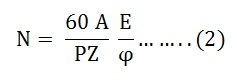# Speed Regulation of DC Motor

If the load is applied to the DC motor or machine, the speed of the motor decreases automatically. Thus to maintain the constant speed, the difference between no-load and full-load speed (called Speed Regulation) should be maintained very less.

The motor is said to be at good regulation if they maintain the constant speed at variable load. The range of the speed regulation of permanent dc motor is from 10% to 15%. If the range is less than 10% then the motor has poor dc regulation. For compound dc motor the regulation range is 25% and for the differential compound motor, it is 5%.

Thus to understand the speed regulation first we should know the speed of the DC Motor.

Contents:

## Speed of a DC Motor

The EMF Equation of a DC motor is given by the equation shown below:Solving for speed of the motor (N) the equation (1) becomes:The above equation (3) shows that the speed of a DC motor is directly proportional to the EMF of rotation E and inversely proportional to the flux per pole.

The expression of EMF of rotation is the same for both motor and generator, equation (2) gives the speed for both the machines.

## Speed Regulation of DC Motor

Definition: The speed regulation of a DC Motor is defined as the change in speed from no load to full load. It is expressed as a fraction or a percentage of the full load speed.

Per unit speed regulation can also be defined as the ratio of the difference between no load to full load with respect to the full load. It is given by the equation shown below:Where,

• Nnl is the no-load speed
• Nfl is the full load speed

A motor which has nearly, constant speed or the difference between no-load and full load is very less is said to have a good speed regulation.